# RBSE Maths Class 8 Chapter 8: Important Questions and Solutions

RBSE Maths Chapter 8 – Visualization of solids Class 8 Important questions and solutions can be accessed here. The important questions and solutions of Chapter 8, available at BYJU’S, contain detailed step by step explanations. The important questions are based on the new design prescribed by the RBSE. Students can also get the syllabus and textbooks on various subjects on RBSE Class 8 solutions.

Chapter 8 of the RBSE Class 8 Maths will help the students to solve problems related to the prism, drawing a triangular prism, pyramid, drawing a soiree pyramid, Euler formula for polyhedrons, 2-dimensional representation of three-dimensional solids: cuboid, cube, cylinder, cone.

### RBSE Maths Chapter 8: Objective Questions and Solutions

Question 1: Plane figures are called

(a) multi-dimensional

(b) three-dimensional

(c) two-dimensional

(d) None of the above

Question 2: Number of vertices in a cuboid is

(a) 12

(b) 8

(c) 6

(d) 4

Question 3: Every solid shape is made up of various two-dimensional figures called

(a) edges

(b) vertices

(c) faces

(d) cuboid

Question 4: Euler’s formula is

(a) F + V = E + 2

(b) F – V = E – 2

(c) V + E = F + 2

(d) V – E = F – 2

Answer: F + V = E + 2

Question 5: The point where the edges of a solid, is called

(a) face

(b) surface

(c) vertex

(d) pyramid

Question 6: An example of a three-dimensional figure is

(a) cuboid

(b) circle

(c) square

(d) rectangle

Question 7: Number of faces in a cuboid is

(a) 6

(b) 8

(c) 12

(d) 10

Question 8: Number of edges in a cube is

(a) 8

(b) 12

(c) 6

(d) 14

Question 9: Number of faces in a cube

(a) 12

(b) 8

(c) 6

(d) 10

### RBSE Maths Chapter 8: Exercise 8.1 Textbook Important Questions and Solutions

Question 1: Fill in the blanks

(i) The base of the pyramid is a polygon and other faces have the ……… shape. (triangle/parallelogram)

(ii) Base face and the top face of a prism are ……… of each other. (congruent/similar)

(iii) A polyhedron whose vertices are 10 and faces are 7 then its edges are ………. (15/19)

(iv) Cube and cuboid are also a kind of ………….. (prism/pyramid)

Solution:

(i) Triangle (ii) Congruent (iii) 15 (iv) Prism

Question 2: Write any four solid polyhedrons surrounding you with the number of its vertices, faces and edges.

Solution:

 Number of vertices Number of faces Number of edges A biscuit packet 8 6 12 Wardrobe 8 6 12 Box 8 6 12 Book 8 6 12

Question 3: Draw a triangular prism taking a 4cm base of an equilateral triangle.

Solution:

Two equilateral triangles of 4cm are taken below at a distance d.

If the corresponding vertices are joined, it results in a triangular prism given below.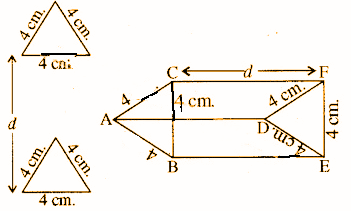Question 4: Recognize polyhedron shapes in the following organised shapes. Also, determine which shapes are used to organise these shapes?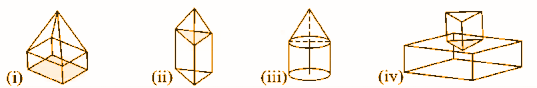Solution:

Figure(i) and (ii) and Polyhedron.

Figure (i) is made by a combination of Cuboid and Pyramid.

Figure (ii) is made by a combination of Prism and Pyramid.

### RBSE Maths Chapter 8: Exercise 8.2 Textbook Important Questions and Solutions

Question 1: Expanding the solid shape of a cube and cuboid, draw different two net patterns other than the ones given in the book.

Solution:

The net pattern of a cube is given below: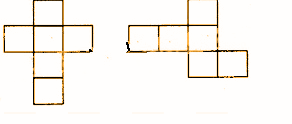The net pattern of a cuboid is given below: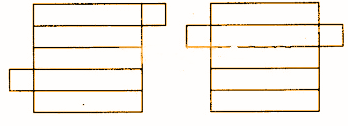Question 2: After cutting the hollow right circular cylinder according to the dotted given in the figure, show the obtained 2d shape.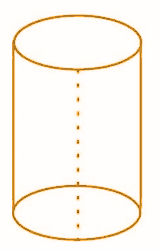Solution: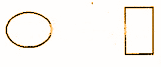Question 3: By folding the expanded figures [by joining the faces], draw the solid figures.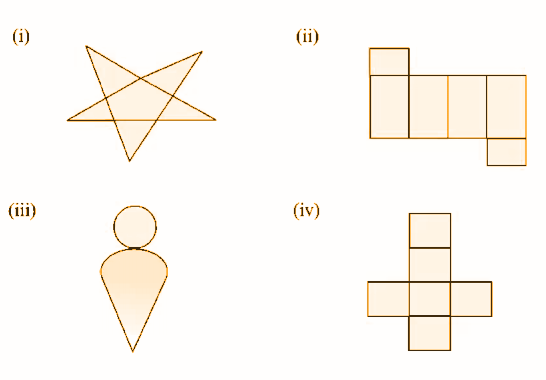Solution: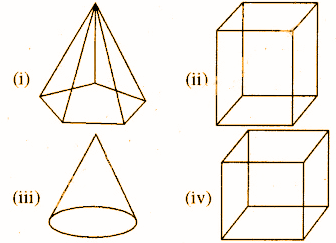### RBSE Maths Chapter 8: Additional Questions and Solutions

Question 1. Find the number of edges and faces in a triangular prism.

Solution:

The number of edges = 9 and

The number of faces = 5.

Question 2: Define two-dimensional shapes.

Solution:

The plane figures consisting of two measurements, length and breadth, are two-dimensional shapes.

Question 3: Give 3 illustrations of two-dimensional shapes.

Solution:

2d shapes: triangle, rectangle circle.

Question 4: Give 3 illustrations of three-dimensional shapes.

Solution:

3d shapes: cuboid, sphere, cylinder.

Question 5. Define a prism.

Solution:

A polyhedron with its base and top are congruent polygons, lateral surfaces are parallelograms called a prism.

Question 6: Define a pyramid.

Solution:

A polyhedron with a polygonal base and triangular sides with a common vertex is called a pyramid.

Question 7: Find the number of faces in the polyhedron having vertices 10 and edges 16.

Solution:

Number of vertices (v) = 10

Number of edges (E) = 16

Number of faces (F) = ?

Euler formula V + F = E + 2

10 + F = 16 + 2

F = 16 + 2 – 10

F = 8

Question 8: Define regular polyhedrons.

Solution:

A regular polyhedron is the one with its faces made of regular polygons and the same faces meet at each of the vertices.

Question 9: Is it possible to have a polyhedron with any given number of faces?

Solution:

Yes, it is possible only if the number of faces is greater than or equal to four.

Question 10: Is a square, prism the same as a cube? Explain.

Solution:

Yes, It can be a cube. It can be a cuboid also.

Question 11: Can a polyhedron have 10 faces, 20 edges and 15 vertices?

Solution:

Since, F + V = E + 2

As 10 + 15 = 20 + 2

A polyhedron cannot have 10 faces, 20 edges and 15 vertices.

Question 12: One vertex of a cube is cut off equidistant from three sides as shown in the figure below. How many faces and vertices will be present in the new figure?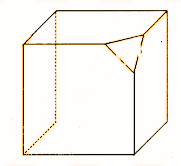Solution:

The number of vertices: 10

The number of faces: 7

Question 13: Can a polyhedron have the following as its faces

(i) 3 triangles?

(ii) 4 triangles?

(iii) a square and four triangles?

Solution:

A polyhedron is a solid which is bounded by four or more polygonal faces in such a way that pairs of faces meet along edges and three or more edges meet in each vertex, hence,

(i) It is not possible that a polyhedron has 3 triangles for its faces.

(ii) 4 triangles can be the faces of a polyhedron.

(iii) A square and 4 triangles can be the faces of a polyhedron.

Question 14: In the following figures, determine which among they are prisms?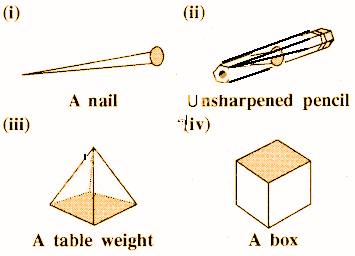Solution:

A prism is a polyhedron whose base and top are congruent polygons and lateral faces are parallelograms. So,

(i) A nail is not a prism.

(ii) An unsharpened pencil is a prism.

(iii) Table weight is not a prism.

(iv) A box is a prism.

Question 15: (i) How are prisms and cylinders alike?

(ii) How are pyramids and cones alike?

Solution:

(i) A prism becomes a cylinder when the number of sides of its base becomes large and larger.

(ii) A pyramid becomes a cone when the number of sides of its base becomes larger and larger.

Question 16: Using Euler’s formula, find the missing numbers.

 Polyhedron Face Vertex Edges A ? 6 12 B 5 ? 9

Solution:

For polyhedron A,

The number of faces =?

The number of vertices = 6,

The number of edges =12

By using Euler’s formula F + V = E + 2 or

F = E + 2 – V = 12 + 2 – 6

F = 14 – 6 = 8

Number of faces F = 8

For polyhedron B,

Number of faces = 5,

Number of vertices = ?,

Number of Edges = 9 ,

By using Euler’s formula F + V = E + 2 or

V = E + 2 – F

= 9 + 2 – 5 = 6 …

Number of vertices = 6

Question 17: Find the number of edges in a polyhedron which has 9 vertices and 9 faces.

Solution:

Number of vertices (V) = 9

Number of faces (F) = 9

Euler’s formula: V+ F = E + 2

9 + 9 = E + 2 or 9 + 9 – 2 = E

So, E = 16

Question 18: In a polyhedron, the number of faces is 5 and the number of edges is 9. Find the number of vertices.

Solution:

Number of faces (F) = 5

Number of Edges (E) = 9

Number of Vertices (V) =?

Euler’s formula F +V = E+ 2

5 + V = 9 + 2

5 + V = 11

V = 11 – 5

V = 6

Number of vertices = 6

Question 19: Fill the table with appropriate answers.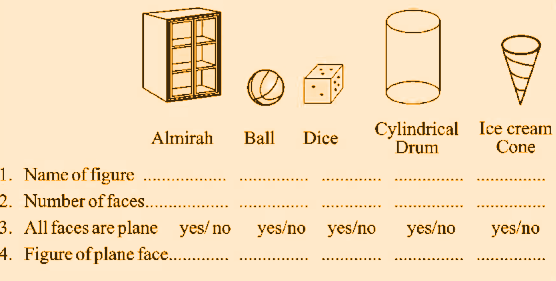Solution:

 Name of the figure Cuboid Sphere Cube Cylinder Cone Number of faces 6 1 6 3 2 Are all faces plane? Yes No Yes No No Shape of plane face Rectangular – Square Circular Circular

Question 20: Fill in the table.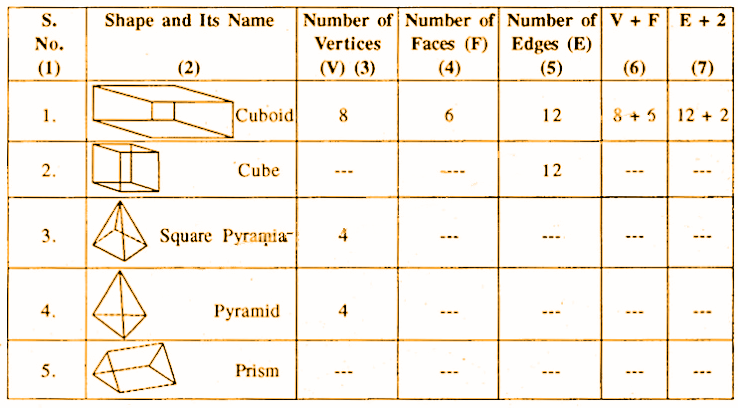Solution:

 Serial no. Name of the shape Number of vertices Number of faces Number of edges V + F E + 2 1 Cuboid 8 6 12 8 + 6 12 + 2 2 Cube 8 8 12 8 + 6 12 + 2 3 Polyhedron 5 5 8 5 + 5 8 + 2 4 Pyramid 4 4 6 4 + 4 6 + 2 5 Prism 6 5 9 6 + 5 9 + 2